Disclaimer: This is an example of a student written essay.

Any opinions, findings, conclusions or recommendations expressed in this material are those of the authors and do not necessarily reflect the views of UKEssays.com.

# Understanding Linear Cryptanalysis

 ✅ Paper Type: Free Essay ✅ Subject: Computer Science ✅ Wordcount: 4885 words ✅ Published: 18th Apr 2018

Dipanjan Bhowmik

Abstract

The objective of this paper is to provide a better understanding of the Linear Cryptanalysis Attack developed by M.Matsui . This paper has been written after going through noted literature in this field and has been structured in such a way that a beginner in this field would be able to understand the idea with little prior knowledge. The paper describes a simple cipher and then applies Linear Cryptanalysis to break it. The cipher has been intentionally taken to be very simple so that a beginner can actually implement it and get an actual feel of the attack. The paper also describes all the algorithms involved in this attack again with the intention of letting a beginner actually realize the attack.

Keywords: Linear Cryptanalysis, Linear Approximation Table, s-box, Toy cipher, Parity.

Introduction:

If one feeds a random input with a particular property into a magic box and can guess the corresponding property in the output, the magic box is some what linear.

For example imagine that the box takes an input and adds one to it. Now, let’s say that the property which is looked at is whether the input/output is even. By feeding it an input, one knows the property will be opposite in the output every single time. In other words, adding one to an even number will always produce an odd number and vice versa. This magic box will be completely linear with respect to divisibility by 2.

In an iterative cipher, substitution box(s) (S-Box(s)) add non linearity to it. Ideally, an s-box should receive an input with property X and output a number that has property Y exactly 50% of the time.

The property, which is being looked at in Linear Cryptanalysis is Parity.

Definition

Parity: It is a Boolean value (a 0 or a 1), that we get if we perform an XOR operation on some or all of the bits of a number expressed in binary form. The bits that are being XORed together is defined by another number called the mask. The mask lets us to ignore some of the bits of the input while calculating the parity. In order to calculate the parity, the mask value it bitwise ANDed with the input value, the bits of the resultant is then taken and XORed together to obtain the parity.

Generating Linear Approximation Tables (LATs):

Algorithm 1: Algorithm for generating Linear Approximation Table

For i=0 to 2m -1

For j=0 to 2n -1

For k=0 to 2m -1

If Parity (k AND i) =Parity(S-box[k] AND j) then

LAT[i][j] ï¦LAT[i][j] +1

Where, LAT is a 2-D array of size m x m.

Parity () is a function that computes the parity of the given input.

M is the total number of bits fed as input to the S-box.

N is the total number of bits produced as output by the S-box.

I ranges from 0 to 2m -1 , it represents all possible input masks.

J ranges from 0 to 2n-1 representing all possible output masks.

K ranges from 0 to 2m -1, it represents all possible inputs to S-box.

Let us assume an S-box that takes 4 bit inputs and produces 4 bit output. Both the input and output ranges from 0 to 15. Such a S-box is injective in nature.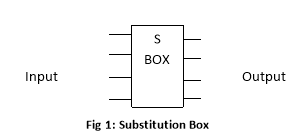I 0 1 2 3 4 5 6 7 8 9 A B C D E F O E 4 D 1 2 F B 8 3 A 6 C 5 9 0 7

For such an S-box, the algorithm to generate the Linear Approximation Table is modified as following:

Algorithm 2: Algorithm for generating Linear Approximation Table for the S-box given in Fig 1.

For i=0 to 15

For j=0 to 15

For k=0 to 15

If Parity (k AND i) =Parity(S-box[k] AND j) then

LAT[i][j]ï¦ LAT[i][j] +1

In this case, the LAT generated is of dimension 16 x 16.The following table depicts the Linear

Approximation Table generated for the S-box given in fig. 1 using algorithm 2.

 Output mask Input mask 0 1 2 3 4 5 6 7 8 9 A B C D E F 0 16 8 8 8 8 8 8 8 8 8 8 8 8 8 8 8 1 8 8 6 6 8 8 6 14 10 10 8 8 10 10 8 8 2 8 8 6 6 8 8 6 6 8 8 10 10 8 8 2 10 3 8 8 8 8 8 8 8 8 10 2 6 6 10 10 6 6 4 8 10 8 6 6 4 6 8 8 6 8 10 10 4 10 8 5 8 6 6 8 6 8 12 10 6 8 4 10 8 6 6 8 6 8 10 6 12 10 8 8 10 8 6 10 12 6 8 8 6 7 8 6 8 10 10 4 10 8 6 8 10 8 12 10 8 10 8 8 8 8 8 8 8 8 8 6 10 10 6 10 6 6 2 9 8 8 6 6 8 8 6 6 4 8 6 10 8 12 10 6 A 8 12 6 10 4 8 10 6 10 10 8 8 10 10 8 8 B 8 12 8 4 12 8 12 8 8 8 8 8 8 8 8 8 C 8 6 12 6 6 8 10 8 10 8 10 12 8 10 8 6 D 8 10 10 8 6 12 8 10 4 6 10 8 10 8 8 10 E 8 10 10 8 6 4 8 10 6 8 8 6 4 10 6 8 F 8 6 4 6 6 8 10 8 8 6 12 6 6 8 10 8

Similarly, the LAT for any of the DES S-box can also be generated, For DES S-box; the algorithm is modified as the following:

Algorithm 3: Algorithm for generating LAT for DES S-Box.

For i=0 to 15

For j=0 to 63

For k=0 to 15

If Parity (k AND i) =Parity(S-box[k] AND j) then

LAT[i][j] ï¦AT[i][j] +1

In this case, the LAT is of dimension 16 x 64, the reason being DES S-box takes 4 bit input and produces 6 bit output.

Piling Up Principle

One of the fundamental tools used for linear cryptanalysis is the Piling Up Principle. Let us conceder two random binary variables X1 and X2, and let us assume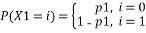And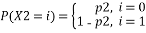Then, the probability of the relationship X1(+)X2 will be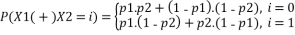That is, X1 (+) X2 will be 0 when X1=X2 i.e. when both X1 and X2 are 0 and both X1 and X2 are 1. And X1 (+) X2 will be 1 when X1≠ X2 i.e. when X1=0 and X2=1 or X1=1 and X2=0. Accordingly probabilities are computed, assuming X1 and X2 are independent.

We are particularly interested in deviation of the probability from ½, so, let us consider p1=1/2+ ε1 and p2=1/2+ε2, where ε1 and ε2 are the deviation of p1 and p2 from respectively from ½ and are referred to as probability bias.

Now, P(X1 (+) X2=0)=(1/2 + ε1).(1/2+ε2) + (1-(1/2+ε1)).(1-(1/2+ε2))

=1/2+2.ε1.ε2

So, probability bias of X1 (+) X2 is given by

ε1,2=2.ε1.ε2

Generally, if X1,X2,…Xn are n independent random binary variables, then the probability of X1 (+) X2 (+) …(+) Xn=0 is given by the Piling Up Lemma.

P( X1 (+) X2 (+) …Xn =0) = ½ + 2 n-1 . ∏i=1…n εi……….(1)

And the probability bias of (+) X2 (+) …(+) Xn=0 is given by

ε1…n=2 n-1 . ∏i=1…n εi

Note that, P( X1 (+) X2 (+) …Xn =0) = ½, if there exist some εi such that εi=0 or pi=1/2. And P( X1 (+) X2 (+) …Xn =0) = 0 or 1, if for all εi, εi=+1/2 or -1/2 respectively or pi=0 or 1 respectively.

Attacking a Toy Cipher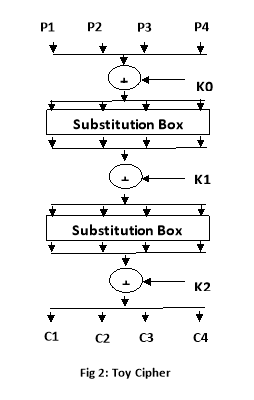Let us consider a toy cipher that takes 4 bit input goes through two iterations of key addition and block substitution and yields a 4 bit output. The following figure diagrammatically represents the toy cipher.

P1, P2, P3, P4 represents the 4 bit plain text

C1, C2, C3, C4 represents 4 bit cipher text.

K0, K1, K2 are 4 bit sub keys

Total key length is of 12 bits.

The cipher uses two identical S-boxes, which is same as the S-box described earlier.

The following algorithm implements the toy cipher

Algorithm 4: Implementing Toy Cipher

Kye[]ï¦{k0,k1,k2}

Sbox[]=ï¦{E,4,D,1,2,F,B,8,A,6,C,5,9,0,7}

For i=0 to 15// 16 possible inputs

{ p=i

For j= 0 to 1// 2 iterations

pï¦Sbox [ p (+) Key[j]]

C[i]ï¦ p (+) Key //final key whitening step

}

The toy cipher yields the following output when Key[]ï¦{B,7,F}

 Plain Text 0 1 2 3 4 5 6 7 8 9 A B C D E F Cipher Text 3 B 6 D 1 7 F 2 4 9 E 5 8 A C 0

The first step towards attacking the cipher begins by obtaining an equation of the form X1 (+) X2 (+)…(+) Xn =0. Such an expression can be obtained using Linear Approximation Table. In our example P(LAT[F][A])=12/16 or equivalently Bias( LAT[F][A})=4/16,k where F is the input mask and A is the output mask. It should be noted that although LAT=16 but it cannot be used.

Let Uij demote the jth input of ith S-Box and Vij denote the jth output of the ith S-Box.

So, P(U11 (+) U12 (+) U13 (+) U14 =V11 (+) V13)= 12/16

Let Kij denote the jth bit of the ith sub key, then U11 = P1 (+) K01, U12 =P2 (+) K02, U13 = P3 (+) K03, and U14 = P4 (+) K04, where Pi denotes the ith plain text bit.

Therefore, P( P1 (+) K01 (+) P2 (+) K02 (+) P3 (+) K03 (+) P4 (+) K04 = V11 (+) V13)) = 12/16

orP ( P1 (+) P2 (+) P3 (+) P4 (+) ∑K0 = V11 (+) V13) = 12/ 16

Since, U21 = V11 (+) K11 or, V11 = U21 (+) K11 and U23 = V23 (+) K13 or, V13 = U23 (+) K13

Hence, P (P1 (+) P2 (+) P3 (+) P4 (+)∑K0 = U21 (+) K11 (+)U23 (+) K13) = 12/ 16

or, P (P1 (+) P2 (+) P3 (+) P4 (+)∑K0 (+) K11 (+) K13 = U21 (+)U23) = 12/ 16

Let us assume K=∑K0 (+) K11 (+) K13, which can either be 0 or 1

Therefore, P (P1 (+) P2 (+) P3 (+) P4 (+) K= U21 (+)U23) = 12/ 16 Or,P (P1 (+) P2 (+) P3 (+) P4 = U21 (+)U23) =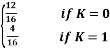Now, as we have obtained a linear expression with a relatively high probability bias, we would now partially decrypt the cipher text to obtain U2 (input to the 2nd S-Box). The following algorithm does this.

Algorithm 5: Partially decrypting the cipher text

C[] ï¦ { 3,B,6,D,1,7,F,2,4,9,E,5,8,A,C,0}

Isbox[] ï¦ {E,3,4,6,1,C,A,F,7,D,9,6,B,2,0,5}

For k=0 to 15

{pro[k]ï¦ 0

For I = 0 to 15

{pdc [k][i] ï¦ isbox [ C[i] (+) k]

If Parity (pdc[k][i] AND A) = Parity ( I AND F) then

pro[k] ï¦ pro[k] +1

}

}

It should be noted that Parity (pdc[k][i] AND A) = Parity ( I AND F) is the algorithmic implementation of P1 (+) P2 (+) P3 (+) P4 (+) = U21 (+) U23. Since, bit wise ANDing retrieves the required bits when ANDed with a mask having 1 in the required position in its binary equivalent.

The algorithm yields the following probabilities.

 Key 0 1 2 3 4 5 6 7 8 9 A B C D E F Probability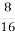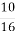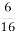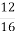From the result we observe that probability when key=F is 12/16 which matches with our expected probability, there by indicating that K2=F.

It should be noted that in our example, it so happened that there is only one candidate for K2, but generally there may be more than one candidate and all of then should be given due consideration.

For the next round, we use the partially decrypted cipher text with respect to key =F as the cipher text and perform the procedure defined as algorithm 5.

That is , now C[]ï¦{B,1,D,4,0,7,E,2,6,A,3,9,F,C,8,5}

The output yielded at this point is given below.

 Key 0 1 2 3 4 5 6 7 8 9 A B C D E F Probability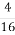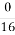At this time we are comparing the plain text block P1, P2, P3, P4 to the input of the first S-Box i.e. U1, U2, U3, U4, so the expected probability is computed as

P( P1 (+) P2 (+) P3 (+) P4 = P1 (+) P2 (+) P3 (+) P4) =1

Or, P( P1 (+) P2 (+) P3 (+) P4 = P1 (+) P2 (+) P3 (+) P4 (+) ∑K0) =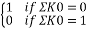Or, P( P1 (+) P2 (+) P3 (+) P4 = P1 (+) K01 (+) P2 (+) K02 (+) P3 (+) K03 (+) P4 (+) K04) =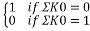Or, P( P1 (+) P2 (+) P3 (+) P4 = U11 (+) U12 (+) U13 (+) U14) =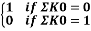The expected probability match4es with the observed probability for sub key K1= 7. Therefore with high degree of certainty, K1=7.

So, we retain the partially decrypted cipher text for sub key =7, which is contained in pdc[i] for i=0 to 15. The partially cipher text for sub key =7 is given in the following table.

 Plain Text 0 1 2 3 4 5 6 7 8 9 A B C D E F Partially decrypted Cipher Text B A 9 8 F E D C 3 2 1 0 7 6 5 4

Now, in order to obtain the sub key K0, we need simply to choose any pair of plain text and partially decrypted cipher text and perform a bitwise XOR operation.

Say, we choose (4,F), then 4 (+) F = B, So, K0=B.

Thus, the actual key ={B, 7, F}, which is the key we originally used in our example toy cipher.

It should be noted that, at every step of our attack, we obtain unique sub key values that matches our expected probability, which may not be the case all the time. And in such situations where multiple sub keys matches the expected probability we need to consider each of these sub keys.

Observations

1. If the Linear Approximation Table (LAT) has an entry such that Bias (LAT[i][j])| =1/2 (50%) and i=j, then the S-box is prone to Linear attack. So, such an S-box is a strict no for any cipher
2. If the Linear Approximation Table has entries such that |Bias(LAT[i][j])| =1/2 and | Bias (LAT[j][k])| = ½ where i ≠ j ≠k , then such a cipher is also susceptible to Linear Attack.
3. If |Bias(LAT[i][j])| = ½ where i≠j and there is no pair such that |Bias(LAT[i][j])|=1/2 and |Bias(LAT[j][k])|=1/2 where i ≠ j ≠k , then after a certain number of iterations, Linear Cryptanalysis becomes ineffective. The observation is illustrated using the following graph.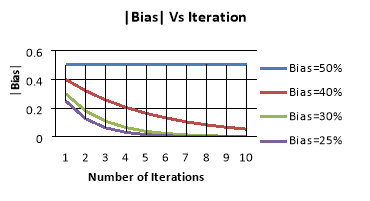Conclusion

As the number of iterations of an iterative cipher increases and observations 1 and 2 does not hold, Linear Cryptanalysis becomes increasingly less effective.

References:

• Heys,H.M,2002,”A Tutorial on Linear And Differential Cryptanalysis”, Cryptologia,XXV(3),189-221.
• Matsui, M.,1994,”Linear Cr4yptanalysis Method For DES Cipher”, Advance in Cryptlogy-EUROCRYPT’93, Springer-Verlag,386-397.
• Jakobson, B.T.,Abyar, M.,Nordholt, P.S.,2006,”Linear And Differential Cryptanalysis”
• Paar, C.,& Pelzl, J.,2010,Understanding Cryptography.Berlin:Springer-Nerlag.

View all

## DMCA / Removal Request

If you are the original writer of this essay and no longer wish to have your work published on UKEssays.com then please:

Related Services

Prices from

KD47

Approximate costs for:

• 1000 words
• 7 day deliveryHumanity University

Dedicated to your worth and value as a human being!

Related Lectures